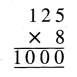# PSEB 4th Class Maths Solutions Chapter 2 Fundamental Operations on Numbers Ex 2.6

Punjab State Board PSEB 4th Class Maths Book Solutions Chapter 2 Fundamental Operations on Numbers Ex 2.6 Textbook Exercise Questions and Answers.

## PSEB Solutions for Class 4 Maths Chapter 2 Fundamental Operations on Numbers Ex 2.6

Question 1.
Cost of 1 notebook is ₹ 15. Find the cost of 9 such notebooks ?
Solution:
Cost of 1 notebook = ₹ 15
Cost of 9 notebooks = ₹ 15 × 9 = ₹ 135.Question 2.
There are 75 pencils in a box. How many pencils are there in 19 such boxes ?
Solution:
Number of pencils in a packet = 75
Number of pencils in 19 packets = 75 × 19
= 1425.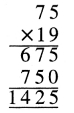Question 3.
There are 79 beads in a chain. How many beads are there in 68 such chains ?
Solution:
Number of beads in one chain = 79
Number of beads in 68 chains = 79 × 68
= 5372.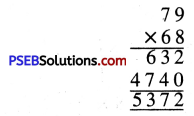Question 4.
The cost of a toycycle is ? 1560. Find total cost of 6 such toycycles ?
Solution:
The cost of 1 toycycle = ₹ 1560
The cost of 6 toycycles = ₹ 1560 × 6
= ₹ 9360.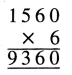Question 5.
There are 11 players in a cricket team. How many players are there in 12 such teams ?
Solution:
Number of players in 1 cricket team = 11
Number of players in 12 cricket teams = 11 × 12 = 132.

Question 6.
A box contains 1440 soaps. Find the numbers of soaps in 6 such boxes ?
Solution:
Number of soaps in 1 box = 1440
Number of soaps in 6 boxes = 1440 × 6
= 8640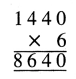Question 7.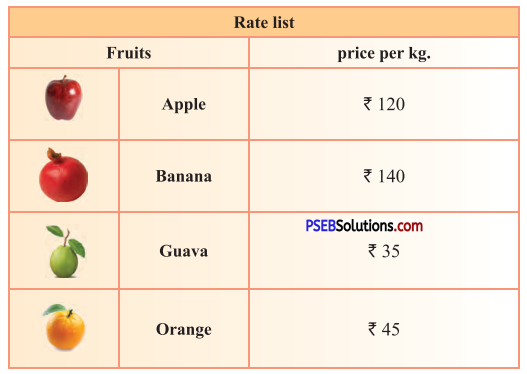(a) She bought 2 kg apples, 2 kg. guava. How much amount she will pay to the seller ?
Solution:
Cost price of 2 kg apples
= ₹ 120 × 2 = ₹ 240
Cost price of 2 kg guava
= ₹ 35 × 2 = ₹ 70
Amount she will pay to the seller = ₹ 310.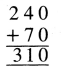(b) If she bought 3 kg oranges and 2 kg pomegranate. What amount she will pay to seller ?
Solution:
Cost price of 3 kg oranges
= ₹ 45 × 3 = ₹ 135
Cost price 2 kg pomegranate
= ₹ 40 × 2 = ₹ 80
Amount she will pay to seller = ₹ 215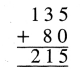Question 8.
These all notes and coins Karan received on his birthday. How much total amount did Karan receive ?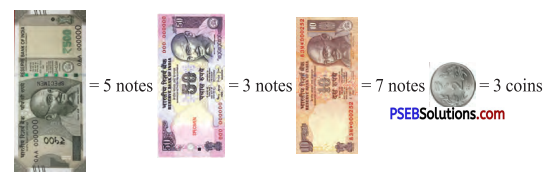Solution:
= ₹ 500 × 5 + ₹ 50 × 3 + ₹ 10 × 7 + ₹ 2 × 3
= ₹ 2500 + ₹ 150 + ₹ 70 + ₹ 6
= ₹ 2726.

Question 9.
A car covers a distance of 16 km in a litre. How much distance it will cover in 28 litres ?
Solution:
Distance covered in 1 litre = 16 km
Distance covered in 28 litres = 16 km × 28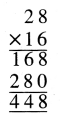= 448 km.Question 10.
A factory produces 125 soap bars in an hour. How many such soap bars will be produced in 8 hours ?
Solution:
Number of soaps produced in 1 hour = 125
Number of soaps produced in 8 hours = 8 × 125
= 1000